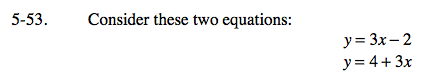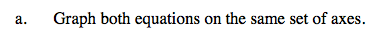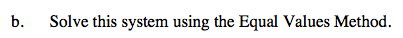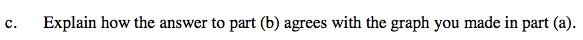### Home > AC > Chapter 5 > Lesson 5.1.5 > Problem5-53

5-53.
1. Consider these two equations: Homework Help ✎

y = 3x − 2

y = 4 + 3x

1. Graph both equations on the same set of axes.

2. Solve this system using the Equal Values Method.

3. Explain how the answer to part (b) agrees with the graph you made in part (a).For help with this problem refer to problem 4-98.